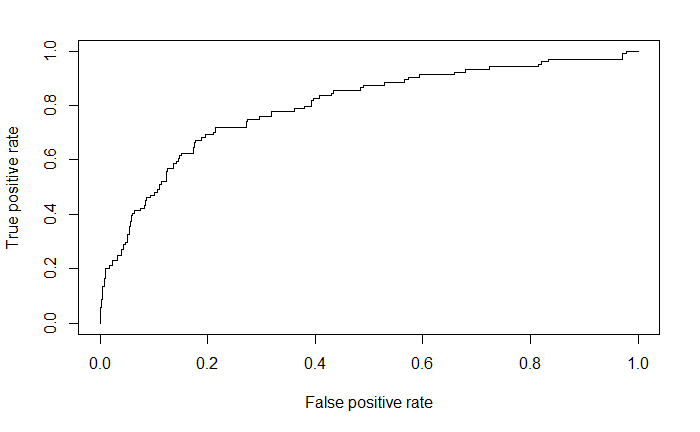//Classification of variables with logistic regression model – GLM## Classification of variables with logistic regression model – GLM

Hello everyone, one of the great dilemmas in all data analysis is to know which are the variables that most influence us in a certain condition.

To know this, a tool that we can use is a logistic regression model: glm.

Logistic regression allows us to know which are the variables that most influence the result of the variable analyzed; therefore, in this post we will make a complete logistic regression model step by step.

The first step is to read the file that we want to analyze, in our case this file is in an Excel format so we have to install the readxl library in R to read it.

setwd(“C:/Users/Sergi/Desktop/Blog de R”)

The formula used in R to perform a logistic regression is glm , before using this function we have to divide the data set in two; train formed by 80% of the total data and in test formed by the remaining 20%. This division is done by a simple random sampling method (more, no_mas).

In the function glm we have to introduce the variable that we want to analyze (in our case Churn) and the rest of variables that influence this variable.

``````set.seed(515616)
mas <- sample(seq(1:nrow(datos)), ceiling(nrow(datos)*0.8))
no_mas <- which(!1:nrow(datos) %in% mas)
train <- datos[mas,]
test <- datos[no_mas,]
modelo <- glm(Churn ~ ., family = binomial(link = 'logit'), data = train)
summary(modelo)``````
``````
Call:
glm(formula = Churn ~ ., family = binomial(link = "logit"), data = train)

Deviance Residuals:
Min      1Q  Median      3Q     Max
-2.119  -0.520  -0.336  -0.188   3.061

Coefficients:
Estimate Std. Error z value Pr(>|z|)
(Intercept)      -5.262230   0.823289  -6.392 1.64e-10 ***
`Day Mins`        0.012578   0.001208  10.413  < 2e-16 ***
`Eve Mins`        0.006385   0.001285   4.970 6.69e-07 ***
`Night Mins`      0.002036   0.001250   1.629   0.1034
`Intl Mins`       0.098624   0.023041   4.280 1.87e-05 ***
`CustServ Calls`  0.435844   0.044124   9.878  < 2e-16 ***
Age              -0.067486   0.005186 -13.013  < 2e-16 ***
`Day Calls`       0.006751   0.003126   2.159   0.0308 *
`Eve Calls`       0.001641   0.003128   0.525   0.5998
`Night Calls`     0.002334   0.003184   0.733   0.4635
---
Signif. codes:  0 *** 0.001 ** 0.01 * 0.05 . 0.1   1

(Dispersion parameter for binomial family taken to be 1)

Null deviance: 2180.4  on 2666  degrees of freedom
Residual deviance: 1692.2  on 2657  degrees of freedom
AIC: 1712.2

Number of Fisher Scoring iterations: 6``````

In the results obtained in the regression we have to focus mainly on two aspects:

• Coeficiente (estimate)
• P-valor (Pr(>|z|)).
To know if a variable is significant or not we only have to look at the p-value because the smaller the p-value, the more significant that variable will be. Given this we find that the significant variabless are `Day Mins``Eve Mins``Intl Mins``CustServ Calls``Day Calls `and Age because they all have a p-value less than 0.1.
The variables that we can not consider as non-significant are `Night Mins``Eve Calls` y `Night Calls `because they have a p-value greater than 0.1.
As for the coefficients, the interpretation changes, if we look at the coefficient of the variable Age (-0.0674486), it is indicating that the probability of leaving the company (Churn) decreases 0.067486 units for each unit that increases the Age variable. & nbsp;

A simpler way to interpret the coefficients is by performing their exponential.
``exp(coefficients(modelo))``
``````     (Intercept)       `Day Mins`       `Eve Mins`     `Night Mins`      `Intl Mins` `CustServ Calls`
0.005183732      1.012657675      1.006405441      1.002037919      1.103651137      1.546267924
Age      `Day Calls`      `Eve Calls`    `Night Calls`
0.934741078      1.006774202      1.001642462      1.002336852 ``````
After performing the exponential coefficients of the model, we obtain that the variables influence the Churn as follows:

• Day Mins: Increase by one unit the variable causes an increase of 0.012% the chances of leaving the company. & nbsp;
• Eve Mins: Increase by one unit the variable causes an increase of 0.006% the chances of leaving the company. & nbsp;
• Night Mins: Increase by one unit the variable causes an increase of 0.002% the chances of leaving the company.
• Intl Mins: Increasing by one unit the variable causes an increase of 0.103% the chances of leaving the company. & nbsp;
• CustServ Calls: Increasing by one unit the variable causes an increase of 0.546% the chances of leaving the company. & nbsp;
• Age: Increasing the variable by one unit causes a 0.07% decrease in the chances of leaving the company. & nbsp;
• Day Calls: Increasing by one unit the variable causes an increase of 0.006% the chances of leaving the company. & nbsp;
• Eve Calls: Increasing by one unit the variable causes an increase of 0.0016% the chances of leaving the company. & nbsp;
• Night Calls: Increasing by one unit the variable causes an increase of 0.0023% the chances of leaving the company.

To know the quality of the model we use the AUC indicator; which can be defined as the probability that a classifier will order a positive instance randomly chosen higher than a negative one. The levels of this indicator are:

• [0.5, 0.6): Bad test. & nbsp;
• [0.6, 0.75): Regular test. & nbsp;
• [0.75, 0.9): Good test. & nbsp;
• [0.9, 0.97): Very good test.
• [0.97, 1): Excellent test.

``library(ROCR)``
``````p <- predict(modelo, test, type = "response")
pr <- prediction(p, test\$Churn)
prf <- performance(pr, measure = "tpr", x.measure = "fpr")
plot(prf)````````````auc <- performance(pr,measure = "auc")
auc <- auc@y.values[]
auc``````

In our case we find an auc = 0.797253: so we can say that our test is good. & nbsp;

We also find the ROC curve which is the representation of true positives against false positives, that is, taking 20% of the sample we would reach 60% of the population.

Greetings. & Nbsp;

SummaryArticle Name
Classification of variables with R
Description
Classification of variables with logistic regression model, One of the great dilemmas in all data analysis is to know which are the variables have influence
Author
Publisher Name
Blog-R
Publisher Logodgedgedg